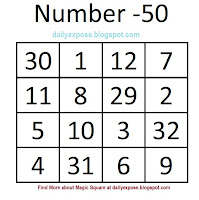### How to Construct Magic Square (Maths Square Secrets)In one of the previous posts we looked about 4 X 4 magic square. In that we have shown a magic square with constant 50. In this post we will find how to construct a magic square.

Using the following steps you can create 4 X 4 magic square with any constant (number) between 22-99.

1. Select a constant number between 22-99 (say Y)
2. Draw a square with 4 rows and 4 columns.

3. First fill these squares (pink squares) using 9, 10, 11, 12 as shown in picture, regardless of the number you have selected.

4. Then fill these squares with 1-8 as shown in picture

5. Now you have to fill only four squares (A, B, C, D)

You can easily calculate A,B,C,D by:
A = Y - 20
B = Y - 19
C = Y - 21
D = Y - 18
where Y is the constant number that you have selected between 22 and 99.
Lets say the constant number Y is 50 then:
A = (50-20) = 30
B = (50-19) = 31
C = (50-21) = 29
D = (50-18) = 32

6. Lets see another example with constant number Y = 55
In this case, A = 35 ,  B=36,  C=34,   D=37

IMPORTANT - If you want to surprise someone with this magic square, don't fill the square using the same steps, which I have shown. If you generate the same way then, they will find the secret easily. So Keep in mind the places where 1-12 should come. Then fill the squares in a random manner. They will say you are a mathematics magician :)